# Content-based Recommender Using Natural Language Processing (NLP)

A guide to build a content-based movie recommender model based on NLP.

By James Ng, Data Science, Project ManagementScreen capture from Netflix

When we provide ratings for products and services on the internet, all the preferences we express and data we share (explicitly or not), are used to generate recommendations by recommender systems. The most common examples are that of Amazon, Google and Netflix.

There are 2 types of recommender systems:

• collaborative filters — based on user rating and consumption to group similar users together, then to recommend products/services to users
• content-based filters — to make recommendations based on similar products/services according to their attributes.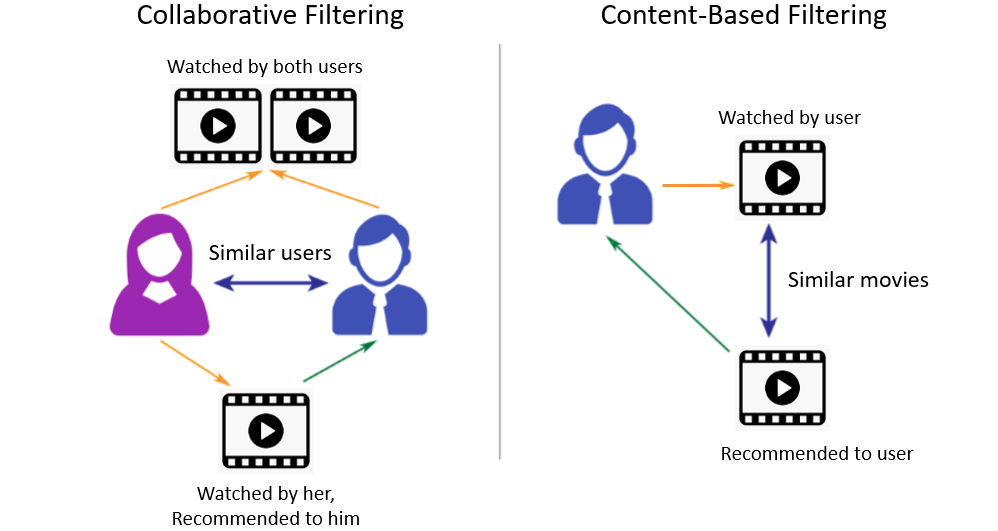In this article, I have combined movie attributes such as genre, plot, director and main actors to calculate its cosine similarity with another movie. The dataset is IMDB top 250 English movies downloaded from data.world.

### Step 1: import Python libraries and dataset, perform EDA

Ensure that the Rapid Automatic Keyword Extraction (RAKE) library has been installed (or pip install rake_nltk).

```from rake_nltk import Rake
import pandas as pd
import numpy as np
from sklearn.metrics.pairwise import cosine_similarity
from sklearn.feature_extraction.text import CountVectorizerdf = pd.read_csv('IMDB_Top250Engmovies2_OMDB_Detailed.csv')

Exploring the dataset, there are 250 movies (rows) and 38 attributes (columns). However, only 5 attributes are useful: ‘Title’, ’Director’, ’Actors’, ’Plot’, and ’Genre’. Below shows a list of 10 popular directors.

`df['Director'].value_counts()[0:10].plot('barh', figsize=[8,5], fontsize=15, color='navy').invert_yaxis()`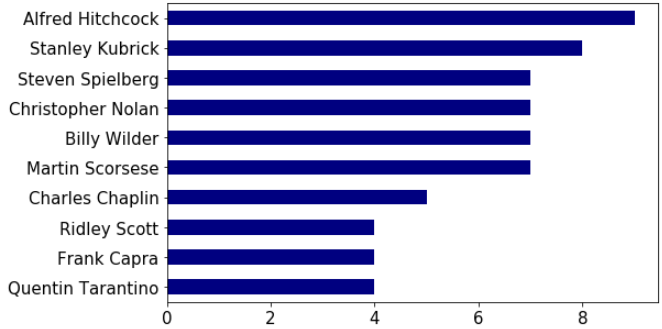Top 10 popular directors amongst the 250 movies

### Step 2: data pre-processing to remove stop words, punctuation, white space, and convert all words to lower case

Firstly the data has to be pre-processed using NLP to obtain only one column that contains all the attributes (in words) of each movie. After that, this information is converted into numbers by vectorization, where scores are assigned to each word. Subsequently cosine similarities can be calculated.

I used the Rake function to extract the most relevant words from whole sentences in the ‘Plot’ column. In order to do this, I applied this function to each row under the ‘Plot’ column and assigned the list of key words to a new column ‘Key_words’.

```df['Key_words'] = ''
r = Rake()for index, row in df.iterrows():
r.extract_keywords_from_text(row['Plot'])
key_words_dict_scores = r.get_word_degrees()
row['Key_words'] = list(key_words_dict_scores.keys())```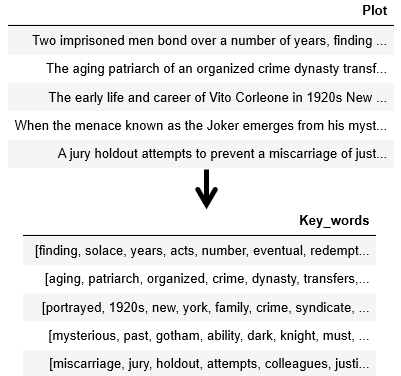Rake function to extract key words

The names of actors and directors are transformed into unique identity values. This is done by merging all first and last names into one word, so that Chris Evans and Chris Hemsworth will appear different (if not, they will be 50% similar because they both have ‘Chris’). Every word needs to be converted to lowercase to avoid duplications.

```df['Genre'] = df['Genre'].map(lambda x: x.split(','))
df['Actors'] = df['Actors'].map(lambda x: x.split(',')[:3])
df['Director'] = df['Director'].map(lambda x: x.split(','))for index, row in df.iterrows():
row['Genre'] = [x.lower().replace(' ','') for x in row['Genre']]
row['Actors'] = [x.lower().replace(' ','') for x in row['Actors']]
row['Director'] = [x.lower().replace(' ','') for x in row['Director']]```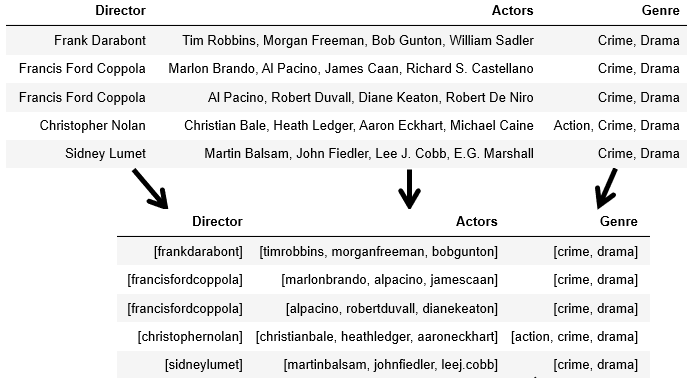All names are transformed into unique identity values

### Step 3: create word representation by combining column attributes to Bag_of_words

After data pre-processing, these 4 columns ‘Genre’, ‘Director’, ‘Actors’ and ‘Key_words’ are combined into a new column ‘Bag_of_words’. The final DataFrame has only 2 columns.

```df['Bag_of_words'] = ''
columns = ['Genre', 'Director', 'Actors', 'Key_words']for index, row in df.iterrows():
words = ''
for col in columns:
words += ' '.join(row[col]) + ' '
row['Bag_of_words'] = words

df = df[['Title','Bag_of_words']]```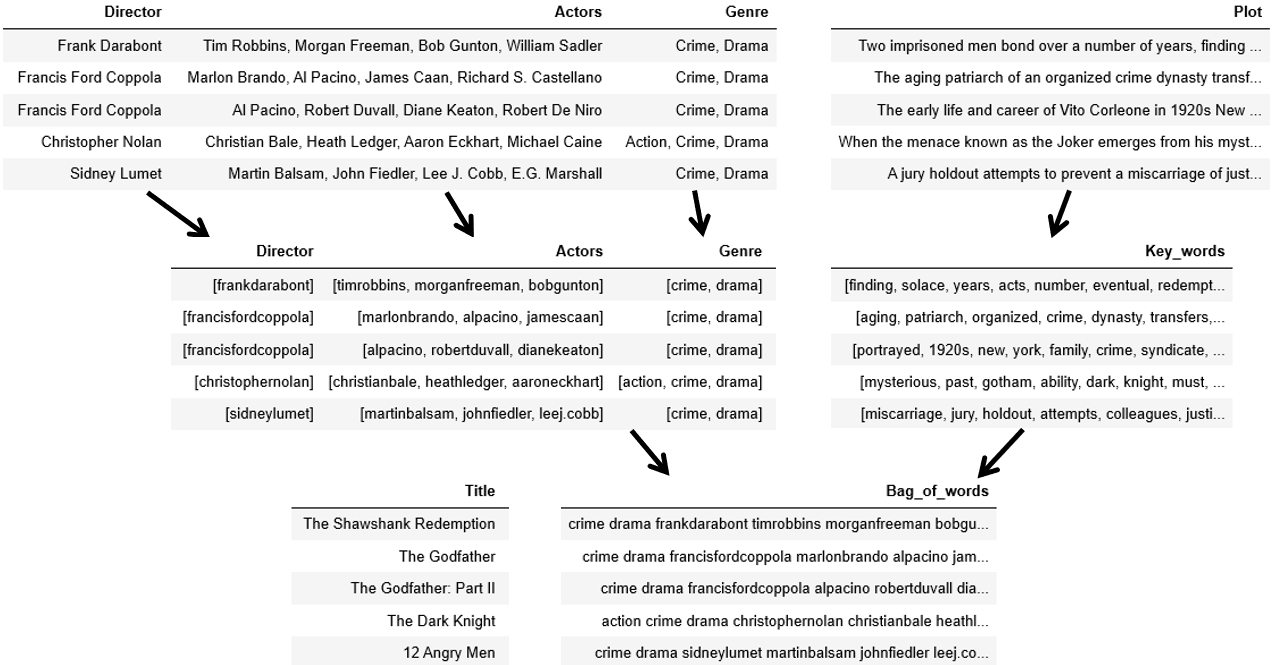Final word representation is the new column ‘Bag_of_words’

### Step 4: create vector representation for Bag_of_words, and create the similarity matrix

The recommender model can only read and compare a vector (matrix) with another, so we need to convert the ‘Bag_of_words’ into vector representation using CountVectorizer, which is a simple frequency counter for each word in the ‘Bag_of_words’ column. Once I have the matrix containing the count for all words, I can apply the cosine_similarity function to compare similarities between movies.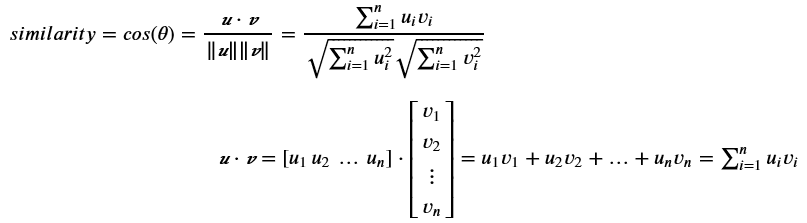Cosine Similarity formula to calculate values in Similarity Matrix

```count = CountVectorizer()
count_matrix = count.fit_transform(df['Bag_of_words'])cosine_sim = cosine_similarity(count_matrix, count_matrix)
print(cosine_sim)```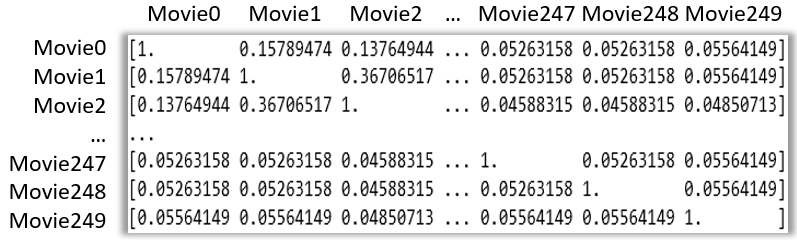Similarity Matrix (250 rows x 250 columns)

Next is to create a Series of movie titles, so that the series index can match the row and column index of the similarity matrix.

`indices = pd.Series(df['Title'])`

### Step 5: run and test the recommender model

The final step is to create a function that takes in a movie title as input, and returns the top 10 similar movies. This function will match the input movie title with the corresponding index of the Similarity Matrix, and extract the row of similarity values in descending order. The top 10 similar movies can be found by extracting the top 11 values and subsequently discarding the first index (which is the input movie itself).

```def recommend(title, cosine_sim = cosine_sim):
recommended_movies = []
idx = indices[indices == title].index
score_series = pd.Series(cosine_sim[idx]).sort_values(ascending = False)
top_10_indices = list(score_series.iloc[1:11].index)

for i in top_10_indices:
recommended_movies.append(list(df['Title'])[i])

return recommended_movies```

Now I am ready to test the model. Let’s input my favourite movie “The Avengers” and see the recommendations.

`recommend('The Avengers')`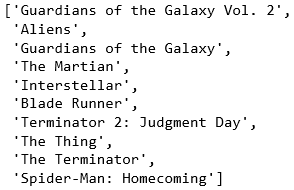Top 10 movies similar to “The Avengers”

### Conclusion

The model has recommended very similar movies. From my “domain knowledge”, I can see some similarities mainly based on directors and plot. I have already watched most of these recommended movies, and am looking forward to watch those few unseen ones.

Python codes with inline comments are available on my GitHub, do feel free to refer to them.